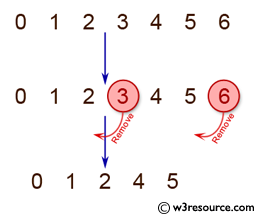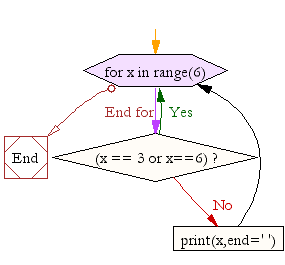﻿ Python Exercise: Prints all the numbers from 0 to 6 except 3 and 6 - w3resource# Python Exercise: Prints all the numbers from 0 to 6 except 3 and 6

## Python Conditional: Exercise-8 with Solution

Write a Python program that prints all the numbers from 0 to 6 except 3 and 6.

Note : Use 'continue' statement.

Pictorial Presentation:Sample Solution:

Python Code:

``````for x in range(6):
if (x == 3 or x==6):
continue
print(x,end=' ')
print("\n")
```
```

Sample Output:

```0 1 2 4 5
```

Flowchart:## Visualize Python code execution:

The following tool visualize what the computer is doing step-by-step as it executes the said program:

Python Code Editor:

Have another way to solve this solution? Contribute your code (and comments) through Disqus.

What is the difficulty level of this exercise?

Test your Python skills with w3resource's quiz

﻿

## Python: Tips of the Day

Returns a list with n elements removed from the beginning

Example:

```def tips_take(itr, n = 1):
return itr[:n]
print(tips_take([1, 2, 3], 5))
print(tips_take([1, 2, 3], 0))
```

Output:

```[1, 2, 3]
[]
```